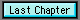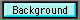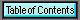Chapter FourAll the Logic You Need to Know

Yesterday I Couldn't Spell It and Today I Are a Web Guru

I really hate this. But ya gotta know how JavaScript logic is specified. Otherwise your scripts can't do much.

The Symbols:

The following symbols are used in JavaScript for comparisons:

 > greater than < less than == equivalent (equal) to != not equivalent (equal) to || or && and ? a:b prior true? if true a, otherwise b

The following symbols are used in JavaScript for actions:

 += a string variable concatenater   (str+="a" same as str=str+"a") += a numeric variable adder   (x+=y is the same as x=x+y) -= a numeric variable subtractor   (x-=y is the same as x=x-y) *= a numeric variable multiplier   (x*=y is the same as x=x*y) /= a numeric variable divider   (x/=y is the same as x=x/y) <<= a numeric bitwise operator   (x<<=y the same as x=x<>= a numeric bitwise operator   (x>>=y the same as x=x>>y) &= a numeric bitwise operator   (x&=y the same as x=x&y) ^= a numeric bitwise operator   (x^=y the same as x=x^y) |= a numeric bitwise operator   (x|=y the same as x=x|y) %= a numeric bitwise operator   (x%=y the same as x=x%y   a modulus is the remainder   of a division of the first variable   by the second)

The following symbols are used in special ways JavaScript for actions:

 x++ a numeric variable incrementer   (x++ is the same as x=x+1)   [If used for setting another value   first returns present value of   x, then increments it) x-- a numeric variable decrementer   (x-- is the same as x=x-1)   [If used for setting another value   first returns present value of   x, then decrements it] ++x a numeric variable incrementer   (first increments the value of   x, then sets variable equal to x) --x a numeric variable decrementer   (first decrements the value of   x, then sets variable equal to x)

If Something, Then Something

To use comparisons of one kind or another in your script, the if-then logic determiner is your tool.

If, for example, the hours in a military time string are greater than 12, you will want your script to subtract 12 from that value to get the time of day in civilian time. (And add a "p.m." as well) How do you do this in JavaScript?

The if comparison takes this form:

if (condition true inside parentheses){
// then do this stuff inside the brackets
}

How does it work in practice?

milhr=15; // milhr set equal to 15
civhr=milhr; // set civhr equal to milhr
civmer="a.m."; // set to am initially
/* OK. Now, if the military hour value is
greater than 12, we want to subtract
12 from the civhr value. Note that
the conditional in the "if" statement
is always put in parentheses. */
if (milhr>12){
civhr=milhr-12;
}
/* But 12 o'clock noon will require a "pm"
so we couldn't include it in the prior "if"
statement. We need a second that
takes account of the 12 as well as all
milhr values greater than 12. */
if (milhr=>12){
civmer="p.m.";
}

The foregoing code can be shortened significantly using the ?a:b format of the if-then conditional.

milhr=15; // milhr set equal to 15
civhr=(milhr>12 ? milhr-12 : milhr);
civmer=(milhr>=12?"p.m.":"a.m.");

Pay particular attention to the double equal sign when making logic statements. If you use the following:

if (a=b){
// then do something here ERROR
}

you will always get an error. The correct form must use the double equal sign to test for equivalence:

if (a==b){
// then do something here CORRECT

}

"If" statements are evaluated by the JavaScript when it executes. If the stuff inside the parentheses evaluates to "true", then the stuff that follows inside the curly brackets is executed. If the stuff inside the parentheses ain't true, it simply skips the stuff inside the curly brackets.

If Something, Then Do Something, Otherwise Do Something Else

This logic is called "If-Then-Else". And all you do is add one more component to the "If-Then" statement.

if (condition true inside parentheses){
// then: do stuff inside these brackets
}
else{
// false: do the stuff in these brackets
}

While Something is True, Keep On Doing Something

The "while" logic allows you to establish a "loop" (in a loop an action is repeated over and over for a number of times)

while (true inside parentheses){
// keep doing stuff in these brackets
}

for (x=y;limit;modifier) Loop

The "for" logic allows you to establish a "loop" that executes a specific number of times.

It has a very rigid and inflexible format. If you deviate from the format, you will get an error.

Within the parentheses are three separate components, separated by semicolons.

The first component within the parentheses is the loop initiator. It sets a loop variable x equal to a value y. This will be a numeric variable.

The second component is the loop limiter. It tells JavaScript when to stop looping.

The third and final component is the variable modifier. Typically it will be a statement that increments or decrements the loop variable.

for (var i=0 ; i<10 ; i++){
// do the stuff inside the curly brackets
}

In the example above, the stuff inside the curly brackets would be executed ten times.

Why? We begin by setting "i" equal to zero. Then, until "i" is greater than 9, we will process the stuff inside the curly brackets. The final component increments "i" by one each time the loop is executed.

Special Note: Exiting from Loops Prematurely

Sometimes you will construct a loop that includes a test to exit or branch prematurely from that loop.

It is advisable to always complete the specified terminal condition in the loop limiter. In the example above, you can terminate the loop properly simply by setting "i" equal to 10.

You may hear about some so-called "memory holes" in browsers. At least some of these are caused by improper loop termination.

A specific memory register is reserved for the loop you create. Until the loop is properly terminated, that register can not be freed up. In iterative code, if you hit that loop again, a new register is reserved, etc. You can run anyone out of memory, given enough iterations.

In particular, many of the "scroller" JavaScripts endemic on the Internet today use exactly that kind of code to continuously require more and more memory for each iteration of the "scroll".

You don't want your visitors to experience the awful, resounding "Oh, No!" of a browser crash.

Order of Analysis - Use of Parentheses

JavaScript reads your code from left to right (just like you do)

This can cause problems with arithmetic sometimes, because JavaScript is very literal and inflexible. For example:

x=7*3+4;
will set
x=25;

x=7*(3+4);
will set
x=49;

So, make sure you use parentheses to group your arithmetical expressions to yield the result you are really looking for.

One further caution: adding strings concatenates them. Adding strings and numerics will yield a concatenated string.

var str="123";
var nr=123;
str=str+nr;
will set
str="123123";